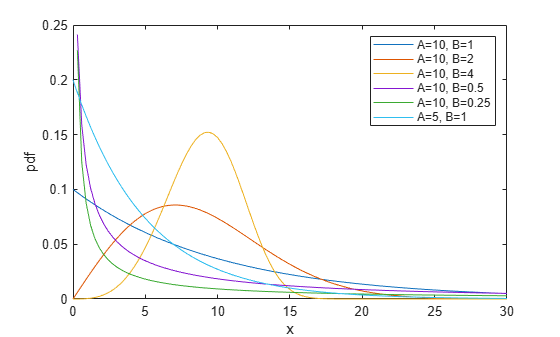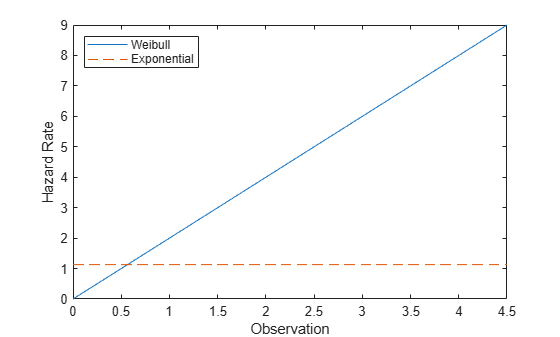## Weibull Distribution

### Overview

The Weibull distribution is a two-parameter family of curves. This distribution is named for Waloddi Weibull, who offered it as an appropriate analytical tool for modeling the breaking strength of materials. Current usage also includes reliability and lifetime modeling. The Weibull distribution is more flexible than the exponential distribution for these purposes, because the exponential distribution has a constant hazard function.

Statistics and Machine Learning Toolbox™ offers several ways to work with the Weibull distribution.

### Parameters

The Weibull distribution uses these parameters.

ParameterDescriptionSupport
`a` Scalea` > 0`
`b`Shapeb` > 0`

The standard Weibull distribution has unit scale.

#### Parameter Estimation

The likelihood function is the probability density function (pdf) viewed as a function of the parameters. The maximum likelihood estimates (MLEs) are the parameter estimates that maximize the likelihood function for fixed values of `x`. The maximum likelihood estimators of a and b for the Weibull distribution are the solution of the simultaneous equations

`$\begin{array}{l}\stackrel{^}{a}={\left[\left(\frac{1}{n}\right)\sum _{i=1}^{n}{x}_{i}^{\stackrel{^}{b}}\right]}^{\frac{1}{\stackrel{^}{b}}}\\ \stackrel{^}{b}=\frac{n}{\left(\frac{1}{\stackrel{^}{a}}\right)\sum _{i=1}^{n}{x}_{i}^{\stackrel{^}{b}}\mathrm{log}{x}_{i}-\sum _{i=1}^{n}\mathrm{log}{x}_{i}}.\end{array}$`

â and $\stackrel{^}{b}$ are unbiased estimators of the parameters a and b.

To fit the Weibull distribution to data and find parameter estimates, use `wblfit`, `fitdist`, or `mle`. Unlike `wblfit` and `mle`, which return parameter estimates, `fitdist` returns the fitted probability distribution object `WeibullDistribution`. The object properties `a` and `b` store the parameter estimates.

For an example, see Fit Weibull Distribution to Data and Estimate Parameters.

### Probability Density Function

The pdf of the Weibull distribution is

`$f\left(x|a,b\right)=\frac{b}{a}{\left(\frac{x}{a}\right)}^{b-1}{e}^{-{\left(x/a\right)}^{b}}.$`

For an example, see Compute Weibull Distribution pdf.

### Cumulative Distribution Function

The cumulative distribution function (cdf) of the Weibull distribution is

`$p=F\left(x|a,b\right)={\int }_{0}^{x}b{a}^{-b}{t}^{b-1}{e}^{-{\left(\frac{t}{a}\right)}^{b}}dt=1-{e}^{-{\left(\frac{x}{a}\right)}^{b}}.$`

The result p is the probability that a single observation from a Weibull distribution with parameters a and b falls in the interval [0 x].

For an example, see Compute Weibull Distribution cdf.

### Inverse Cumulative Distribution Function

The inverse cdf of the Weibull distribution is

`$x={F}^{-1}\left(p|a,b\right)=-a{\left[\mathrm{ln}\left(1-p\right)\right]}^{1/b}.$`

The result x is the value where an observation from a Weibull distribution with parameters a and b falls in the range [0 x] with probability p.

### Hazard Function

The hazard function (instantaneous failure rate) is the ratio of the pdf and the complement of the cdf. If f(t) and F(t) are the pdf and cdf of a distribution, then the hazard rate is $h\left(t\right)=\frac{f\left(t\right)}{1-F\left(t\right)}$. Substituting the pdf and cdf of the exponential distribution for f(t) and F(t) above yields the function $\frac{b}{{a}^{b}}{x}^{b-1}$.

For an example, see Compare Exponential and Weibull Distribution Hazard Functions.

### Examples

#### Fit Weibull Distribution to Data and Estimate Parameters

Simulate the tensile strength data of a thin filament using the Weibull distribution with the scale parameter value `0.5` and the shape parameter value `2`.

```rng('default'); % For reproducibility strength = wblrnd(0.5,2,100,1); % Simulated strengths```

Compute the MLEs and confidence intervals for the Weibull distribution parameters.

`[param,ci] = wblfit(strength)`
```param = 1×2 0.4768 1.9622 ```
```ci = 2×2 0.4291 1.6821 0.5298 2.2890 ```

The estimated scale parameter is `0.4768`, with the 95% confidence interval `(0.4291,0.5298)`.

The estimated shape parameter is `1.9622`, with the 95% confidence interval `(1.6821,2.2890)`.

The default confidence interval for each parameter contains the true value.

#### Compute Weibull Distribution pdf

Compute the pdf of the Weibull distribution with the scale parameter value `3` and the shape parameter value `2`.

```x = 0:0.1:10; y = wblpdf(x,3,2);```

Plot the pdf.

```figure; plot(x,y) xlabel('Observation') ylabel('Probability Density')```#### Compute Weibull Distribution cdf

Compute the cdf of the Weibull distribution with the scale parameter value `3` and the shape parameter value `2`.

```x = 0:0.1:10; y = wblcdf(x,3,2);```

Plot the cdf.

```figure; plot(x,y) xlabel('Observation') ylabel('Cumulative Probability')```#### Compare Exponential and Weibull Distribution Hazard Functions

The exponential distribution has a constant hazard function, which is not generally the case for the Weibull distribution. In this example, the Weibull hazard rate increases with age (a reasonable assumption).

Compute the hazard function for the Weibull distribution with the scale parameter value `1` and the shape parameter value `2`.

```t = 0:0.1:4.5; h1 = wblpdf(t,1,2)./(1-wblcdf(t,1,2));```

Compute the mean of the Weibull distribution with scale parameter value `1` and shape parameter value `2`.

`mu = wblstat(1,2)`
```mu = 0.8862 ```

Compute the hazard function for the exponential distribution with mean `mu`.

`h2 = exppdf(t,mu)./(1-expcdf(t,mu));`

Plot both hazard functions on the same axis.

```plot(t,h1,'-',t,h2,'--') xlabel('Observation') ylabel('Hazard Rate') legend('Weibull','Exponential','location','northwest')```#### Estimate Parameters of Three-Parameter Weibull Distribution

Statistics and Machine Learning Toolbox™ uses a two-parameter Weibull distribution with a scale parameter $a$ and a shape parameter $b$. The Weibull distribution can take one more parameter, a location parameter $c$. The pdf becomes

where $a$ and $b$ are positive values, and $c$ is a real value.

Generate sample data of size 1000 from a three-parameter Weibull distribution with the scale parameter 1, shape parameter 1, and location parameter 10.

```rng('default') % For reproducibility data = wblrnd(1,1,[1000,1]) + 10;```

Define a probability density function for a three-parameter Weibull distribution.

`custompdf = @(x,a,b,c) (x>c).*(b/a).*(((x-c)/a).^(b-1)).*exp(-((x-c)/a).^b);`

`mle` estimates the parameters from data. If `mle` does not converge with default statistics options, modify them by using the name-value pair argument `'Options'`.

Create a statistics options structure `opt` by using the function `statset`.

`opt = statset('MaxIter',1e5,'MaxFunEvals',1e5,'FunValCheck','off');`

The option `opt` includes the following options:

• `'MaxIter',1e5` — Increase the maximum number of iterations to `1e5`.

• `'MaxFunEvals',1e5` — Increase the maximum number of object function evaluations to `1e5`.

• `'FunValCheck','off'` — Turn off checking for invalid object function values.

For a distribution with a region that has zero probability density, `mle` might try some parameters that have zero density, and it will fail to estimate parameters. To avoid this problem, you can turn off the option that checks for invalid function values by using `'FunValCheck','off'`.

Use `mle` to estimate the parameters. Note that the Weibull probability density function is positive only for $x>c$. This constraint also implies that a location parameter $c$ is smaller than the minimum of the sample data. Include the lower and upper bounds of parameters by using the name-value pair arguments `'LowerBound'` and `'UpperBound'`, respectively.

```params = mle(data,'pdf',custompdf,'start',[5 5 5],... 'Options',opt,'LowerBound',[0 0 -Inf],'UpperBound',[Inf Inf min(data)])```
```params = 1×3 1.0258 1.0618 10.0004 ```

If the scale parameter $b$ is smaller than 1, the probability density of the Weibull distribution approaches infinity as $x$ goes to $c$, where $c$ is the location parameter. The maximum of the likelihood function is infinite. `mle` may find satisfactory estimates in some cases, but the global maximum is degenerate when $b<1$.

### Related Distributions

• Exponential Distribution — The exponential distribution is a one-parameter continuous distribution that has parameter μ (mean). This distribution is also used for lifetime modeling. When b = 1, the Weibull distribution is equal to the exponential distribution with mean μ = a.

• Extreme Value Distribution — The extreme value distribution is a two-parameter continuous distribution with parameters µ (location) and σ (scale). If X has a Weibull distribution with parameters a and b, then log X has an extreme value distribution with parameters µ = log a and σ = 1/b. This relationship is used to fit data to a Weibull distribution.

• Rayleigh Distribution — The Rayleigh distribution is a one-parameter continuous distribution that has parameter b (scale). If A and B are the parameters of the Weibull distribution, then the Rayleigh distribution with parameter b is equivalent to the Weibull distribution with parameters$A=\sqrt{2}b$ and B = 2.

• Three-Parameter Weibull Distribution — The three-parameter Weibull distribution adds a location parameter that is zero in the two-parameter case. If X has a two-parameter Weibull distribution, then Y = X + c has a three-parameter Weibull distribution with the added location parameter c.

For an example, see Estimate Parameters of Three-Parameter Weibull Distribution.

 Crowder, Martin J., ed. Statistical Analysis of Reliability Data. Reprinted. London: Chapman & Hall, 1995.

 Devroye, Luc. Non-Uniform Random Variate Generation. New York, NY: Springer New York, 1986. https://doi.org/10.1007/978-1-4613-8643-8

 Evans, Merran, Nicholas Hastings, and Brian Peacock. Statistical Distributions. 2nd ed. New York: J. Wiley, 1993.

 Lawless, Jerald F. Statistical Models and Methods for Lifetime Data. 2nd ed. Wiley Series in Probability and Statistics. Hoboken, N.J: Wiley-Interscience, 2003.

 Meeker, William Q., and Luis A. Escobar. Statistical Methods for Reliability Data. Wiley Series in Probability and Statistics. Applied Probability and Statistics Section. New York: Wiley, 1998.